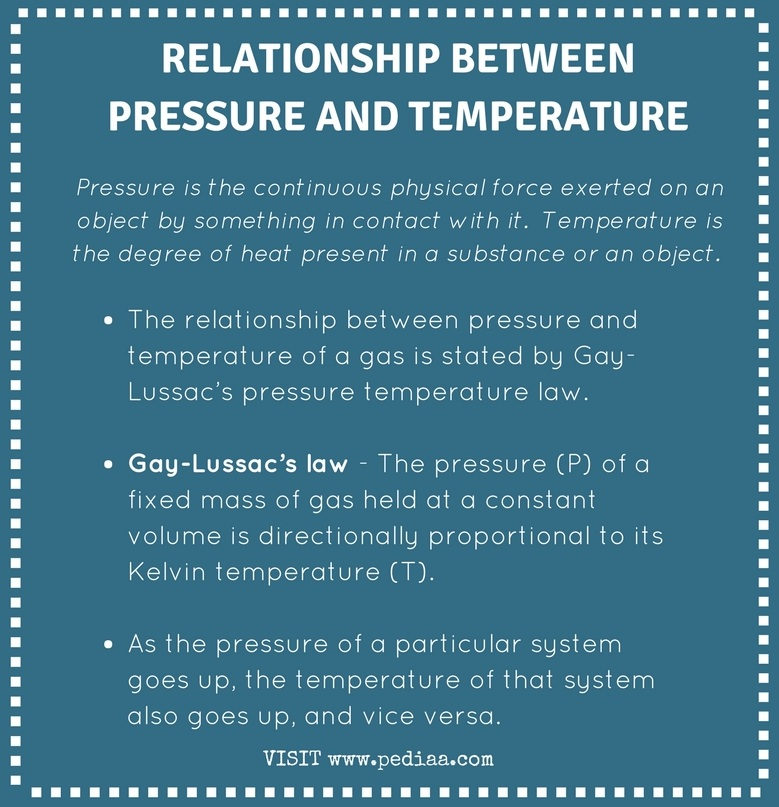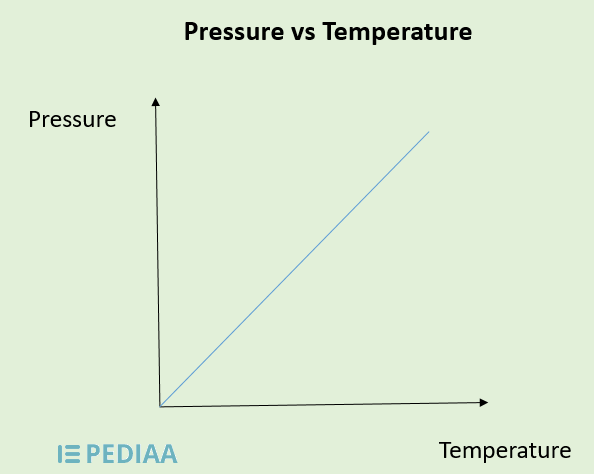# Relationship Between Pressure and Temperature

The relationship between pressure and temperature of a gas is stated by Gay-Lussac’s pressure temperature law. This law states that the pressure (P) of a fixed mass of gas held at a constant volume is directionally proportional to its Kelvin temperature (T). Therefore, as the pressure of a particular system goes up, the temperature of that system also goes up, and vice versa. Gas laws describe the behavior of gases with respect to the pressure, volume, temperature, and amount. Gases are one of the states of matter, either compressed very tightly or expanded to fill a large space.

### Key Areas Covered

1. What is Pressure
– Definition, Pressure of a Gas
2. What is Temperature
– Definition, Measurement
3. What is the Relationship Between Pressure and Temperature
– Gay-Lussac’s Law

Key Terms: Gas, Gay-Lussac’s Law, Kelvin, Pressure, Temperature## What is Pressure

Pressure is a continuous physical force exerted on an object by something in contact with it. It is calculated as the force per unit area. When an enclosed chamber of gas surrounded by a vacuum is considered, the pressure exerted by the gas on the walls of the chamber depends on three factors. They are the amount of gas in the chamber, the volume of the chamber, and the temperature of the gas. When the other parameters are constant, the pressure inside the chamber is directly proportional to the amount of gas in the chamber; it is inversely proportional to the volume of the chamber; it is directly proportional to the temperature of the gas inside the chamber. Pressure is defined in figure 1.Figure 1: Pressure

The atmospheric pressure is created by the weight of air above us. At sea level, it is 105 Pa.

## What is Temperature

Temperature is the degree of heat present in a substance or an object. It represents internal energy contained within a particular system. Temperature can be measured with a thermometer, which is calibrated in various units of measurement. Celsius scale is the most widely used scale for measuring temperature, which is denoted in °C. The unit of temperature based on the International System of Units (SI) is the Kelvin (K). A thermometer is shown in figure 2.Figure 2: Thermometer

At the coldest theoretical temperature, which is absolute zero, the thermal motion of particles in a matter is minimum. The absolute zero is 0 K, and it is -273.14 °C.

## What is the Relationship Between Pressure and Temperature

The relationship between pressure and temperature is described with respect to gases. Gay-Lussac’s law is the gas law that describes the pressure-temperature relationship. It states that, at a constant volume, the pressure of a given amount of a particular gas is directly proportional to its Kelvin temperature. It can be written as:

• P ∝ T, or
• P/T = k where k is a constant, or
• P1/T1 = P2/T2Figure 3: Relationship Between Pressure and Temperature

When the temperature of a particular system is increased, the molecules in the gas move faster, exerting a greater pressure on the wall of the gas container. This in terms increases the pressure of the system. If the temperature of the system is decreased, the pressure goes down. Therefore, at a constant volume, the pressure of a particular gas is directly proportional to the temperature.

### Conclusion

The pressure of a given amount of gas is directly proportional to the temperature at a given volume. When the temperature of a system goes up, the pressure also goes up, and vice versa. The relationship between pressure and temperature of a gas is stated by the Gay-Lussac’s law.

##### Reference:

1. “9.2 Relating Pressure, Volume, Amount, and Temperature: The Ideal Gas Law.” Chemistry, Available here.

##### Image Courtesy:

1. “Pressure force area” By Klaus-Dieter Keller – Own work (CC BY-SA 3.0) via Commons Wikimedia
2. “1134182” (CC0) via Pixabay### About the Author: Lakna

Lakna, a graduate in Molecular Biology & Biochemistry, is a Molecular Biologist and has a broad and keen interest in the discovery of nature related things